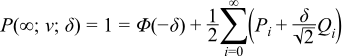#Boost C++ Libraries

...one of the most highly regarded and expertly designed C++ library projects in the world.

This is the documentation for an old version of boost. Click here for the latest Boost documentation.
##### Noncentral T Distribution

`#include <boost/math/distributions/non_central_t.hpp>`

```namespace boost{ namespace math{

template <class RealType = double,
class Policy   = policies::policy<> >
class non_central_t_distribution;

typedef non_central_t_distribution<> non_central_t;

template <class RealType, class Policy>
class non_central_t_distribution
{
public:
typedef RealType  value_type;
typedef Policy    policy_type;

// Constructor:
non_central_t_distribution(RealType v, RealType delta);

// Accessor to degrees_of_freedom parameter v:
RealType degrees_of_freedom()const;

// Accessor to non-centrality parameter lambda:
RealType non_centrality()const;
};

}} // namespaces
```

The noncentral T distribution is a generalization of the Students t Distribution. Let X have a normal distribution with mean δ and variance 1, and let ν S2 have a chi-squared distribution with degrees of freedom ν. Assume that X and S2 are independent. The distribution of tν(δ)=X/S is called a noncentral t distribution with degrees of freedom ν and noncentrality parameter δ.

This gives the following PDF:where 1F1(a;b;x) is a confluent hypergeometric function.

The following graph illustrates how the distribution changes for different values of δ:##### Member Functions
```non_central_t_distribution(RealType v, RealType lambda);
```

Constructs a non-central t distribution with degrees of freedom parameter v and non-centrality parameter delta.

Requires v > 0 and finite delta, otherwise calls domain_error.

```RealType degrees_of_freedom()const;
```

Returns the parameter v from which this object was constructed.

```RealType non_centrality()const;
```

Returns the non-centrality parameter delta from which this object was constructed.

##### Non-member Accessors

All the usual non-member accessor functions that are generic to all distributions are supported: Cumulative Distribution Function, Probability Density Function, Quantile, Hazard Function, Cumulative Hazard Function, mean, median, mode, variance, standard deviation, skewness, kurtosis, kurtosis_excess, range and support.

The domain of the random variable is [-∞, +∞].

##### Accuracy

The following table shows the peak errors (in units of epsilon) found on various platforms with various floating-point types. Unless otherwise specified, any floating-point type that is narrower than the one shown will have effectively zero error.

Table 15. Errors In CDF of the Noncentral T Distribution

Significand Size

Platform and Compiler

ν,δ < 600

53

Win32, Visual C++ 8

Peak=120 Mean=26

64

RedHat Linux IA32, gcc-4.1.1

Peak=121 Mean=26

64

Redhat Linux IA64, gcc-3.4.4

Peak=122 Mean=25

113

HPUX IA64, aCC A.06.06

Peak=115 Mean=24

CautionThe complexity of the current algorithm is dependent upon δ2: consequently the time taken to evaluate the CDF increases rapidly for δ > 500, likewise the accuracy decreases rapidly for very large δ.

Accuracy for the quantile and PDF functions should be broadly similar, note however that the mode is determined numerically and can not in principal be more accurate than the square root of machine epsilon.

##### Tests

There are two sets of tests of this distribution: basic sanity checks compare this implementation to the test values given in "Computing discrete mixtures of continuous distributions: noncentral chisquare, noncentral t and the distribution of the square of the sample multiple correlation coefficient." Denise Benton, K. Krishnamoorthy, Computational Statistics & Data Analysis 43 (2003) 249-267. While accuracy checks use test data computed with this implementation and arbitary precision interval arithmetic: this test data is believed to be accurate to at least 50 decimal places.

##### Implementation

The CDF is computed using a modification of the method described in "Computing discrete mixtures of continuous distributions: noncentral chisquare, noncentral t and the distribution of the square of the sample multiple correlation coefficient." Denise Benton, K. Krishnamoorthy, Computational Statistics & Data Analysis 43 (2003) 249-267.

This uses the following formula for the CDF:Where Ix(a,b) is the incomplete beta function, and Φ(x) is the normal CDF at x.

Iteration starts at the largest of the Poisson weighting terms (at i = δ2 / 2) and then proceeds in both directions as per Benton and Krishnamoorthy's paper.

Alternatively, by considering what happens when t = ∞, we have x = 1, and therefore Ix(a,b) = 1 and:From this we can easily show that:and therefore we have a means to compute either the probability or its complement directly without the risk of cancellation error. The crossover criterion for choosing whether to calculate the CDF or it's complement is the same as for the Noncentral Beta Distribution.

The PDF can be computed by a very similar method using:Where Ix'(a,b) is the derivative of the incomplete beta function.

The quantile is calculated via the usual derivative-free root-finding techniques, with the initial guess taken as the quantile of a normal approximation to the noncentral T.

There is no closed form for the mode, so this is computed via functional maximisation of the PDF.

The remaining functions (mean, variance etc) are implemented using the formulas given in Weisstein, Eric W. "Noncentral Student's t-Distribution." From MathWorld--A Wolfram Web Resource. http://mathworld.wolfram.com/NoncentralStudentst-Distribution.html and in the Mathematica documentation.

Some analytic properties of noncentral distributions (particularly unimodality, and monotonicity of their modes) are surveyed and summarized by:

Andrea van Aubel & Wolfgang Gawronski, Applied Mathematics and Computation, 141 (2003) 3-12.

 Copyright © 2006 , 2007, 2008, 2009 John Maddock, Paul A. Bristow, Hubert Holin, Xiaogang Zhang, Bruno Lalande, Johan Råde, Gautam Sewani and Thijs van den Berg Distributed under the Boost Software License, Version 1.0. (See accompanying file LICENSE_1_0.txt or copy at http://www.boost.org/LICENSE_1_0.txt)